# Deciding where to drop frames algorithmically

Hey all, this is my first post on the dev forums. Nice to meet you.

This is more of a conceptual problem than an actual scripting problem, so I’m going to refrain from giving code examples and get to what my actual problem is.

I am working on a game that handles all of its logic through scripts on a frame-by-frame basis. It is absolutely essential that this logic is run through close to 60 times a second to keep the intended speed of the game, so I have it programmed it to only handle rendering updates on certain frames (while the logic is performed every frame). This helps free up the workload and maintain a near-consistent frame rate even on lower machines.

The way I do this is by performing a check every RenderStepped. If the frame rate is below 55, I will lower the amount of render updates by 1. This will lead to the game lowering the rate of render updates until the game reaches a stable frame rate.

Currently, the way I decide how to drop render updates is by taking 60 and diving it by the number of missing render frames. Let’s call this variable “firstSplit”. Then, if frame % math.ceil(firstSplit) == 0, rendering will not be performed on that frame.
This works well because it evenly distributes where dropped render updates will be placed, keeping the game as smooth as possible.

Here are some images to visualize this technique:
Gray bars are frames where both logic and rendering are performed.
Red bars are frames where only logic is performed (frames with no render updates)

60 renders a second (perfect performance)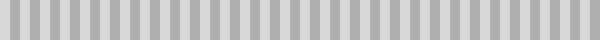59 renders a second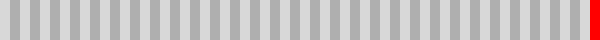58 renders a second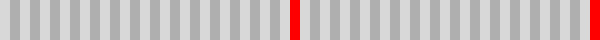57 renders a second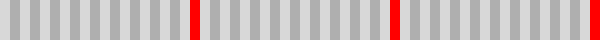56 renders a second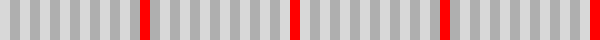And here is 30 renders per second.

The problem with going below 30 is my method doesn’t work below that. Does anybody know the math to perform this same type of operation below 30? Let me know in the replies, or if you have any alternative approaches to handling this type of problem, I’d be game to hear that as well.

1 Like

``````local frames = 60
local dropped = 37
local rendered = frames-dropped
local steprate = rendered/frames

local result = ""

for i = 1, frames do
if math.floor(i*steprate) == math.floor((i+1)*steprate) then
result = result.."x"
else
result = result.."|"
end
end

print(result)
``````

Here x represents a dropped frame.

dropped = 5: |||||||||||x|||||||||||x|||||||||||x|||||||||||x|||||||||||x
dropped = 27: |x|x|x|x||x|x|x|x||x|x|x|x|x||x|x|x|x||x|x|x|x|x||x|x|x|x||x
dropped = 47: xxx|xxxx|xxx|xxxx|xxxx|xxx|xxxx|xxx|xxxx|xxxx|xxx|xxxx|xxx|x

What I do is I have a step rate - which is the number of frames you render divided by the total number of frames you have. By calling `math.floor(i*steprate)` you can find which frame number has to be drawn at interval i. If that number is the same as the number you get for the next interval (i+1) then it means you don’t have to update for the next frame so you can ‘drop’ your current one. This should work for any number between 1 and 60, so if you find any issues let me know.

Also, hi! Welcome to the forum.

5 Likes

As an alternative to spiking computation and trying to determine the best places to perform these spikes, I’d look at smoothing out the computations. Can the updates you are doing overflow into the next frame if they take too long? Pausing updates a little sooner rather than stopping two whole frames to perform logic would be much less noticeable. Out of curiosity, what is requiring so much performance? Generally performance heavy calculations should be added to the engine so compiled C++ can handle it. If it should be done in Lua, it could help make a case for LuaJIT. ^.^

1 Like

Thanks! This works perfectly.

Can the updates you are doing overflow into the next frame if they take too long?

They cannot, because every frame is dependent on the updates that occurred in the frame before it. I actually have a control in place just to ensure all the code from one step has completed running before allowing a new step to take place.

what is requiring so much performance?

All collisions and position data is handled in the game logic. What’s conveyed in the actual workspace is really just a visual representation of what’s being handled in my own code.

Generally performance heavy calculations should be added to the engine so compiled C++ can handle it.

Could you send me some reading/videos on this? I wasn’t aware Roblox let you do this type of thing.

Here is some footage of the game in motion (note: this video was uploaded by another user so it may be taken down at any time)